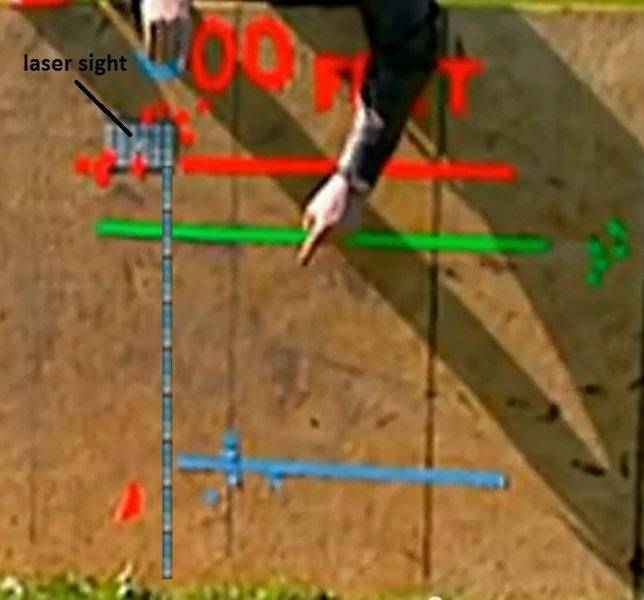# Air resistance on a bullet

• muppet

#### muppet

In this clip the mythbusters are investigating projectile motion at a firing range:

Using the fact that the bullet fell approximately 1 inch as it traveled 100 feet, I predicted it should travel 600 feet down the firing range before hitting the ground from a height of 36 inches. Instead, it actually traveled about 360 feet.

Am I right in assuming that this discrepancy is due to air resistance, and that the simplifying assumption of neglecting it is just way off in this case? I'm trusting their rough numbers here, but even if I double the height through which the bullet fell in the 1st 100 feet I still predict it should travel 450 ft before hitting the ground.

Am I right in assuming that this discrepancy is due to air resistance, and that the simplifying assumption of neglecting it is just way off in this case?
Yes, assuming you simply used the basic kinematic equations, you're right. Air resistance is not negligible in almost all applications involving fast motion through atmosphere. The reason it's routinelly left our from introductory physics problem analysis is that requires solving a (simple one, but still) differential equation.

•muppet
OK, thanks. Just checking- I've never actually studied a real-life example of projectile motion, rather than one with made-up numbers before!

They only tell you the drop distance for the 100 foot distance. If they had stated the actual drop distances for 200 and 300 feet, you would be able to see how the bullet is slowing down as it travels downrange.

Here's a screenshot of the board. I've marked the laser sight position that's centered on the white card. I pasted 9 copies of one column to verify that the sight is 36 inches from the bottom. The last pasted column is about two markings above the ground. Combined with the two markings that the laser sight was from the bottom of the card, there are 40 units of measure from the laser sight to the ground. Assuming that they are one inch markings, then they sighted in at 40 inches and the bullets at 100 feet were closer to having a 2 inch drop. Even if you accept the 36 inch sighting statement, the first drop is still closer to 2 inches.

Long story short: The first drop was closer to two inches. :)